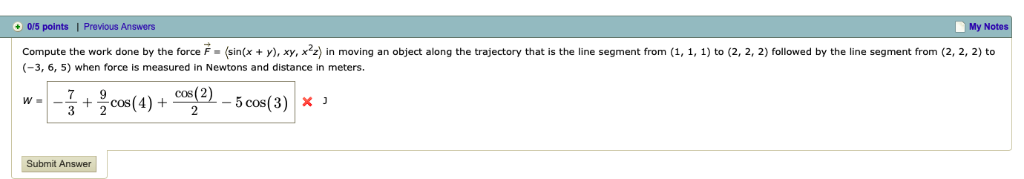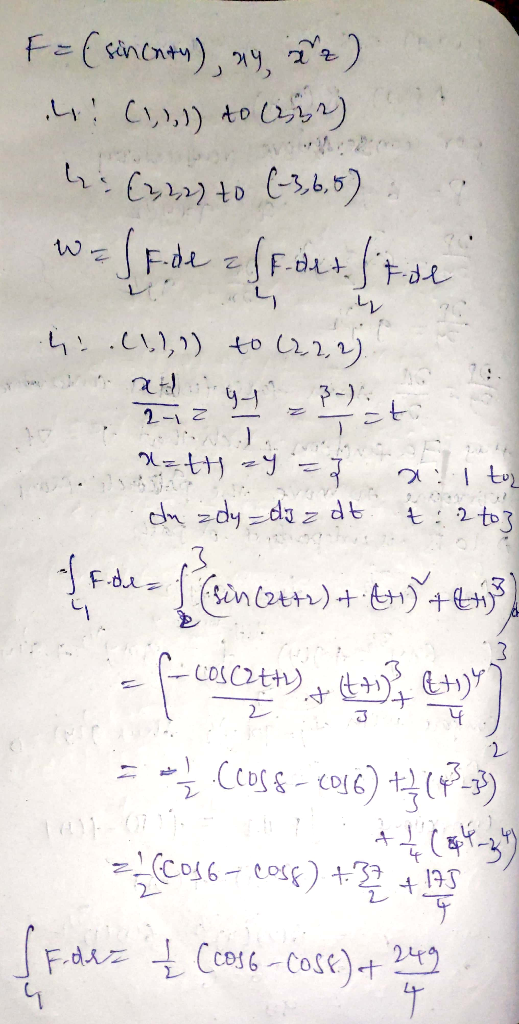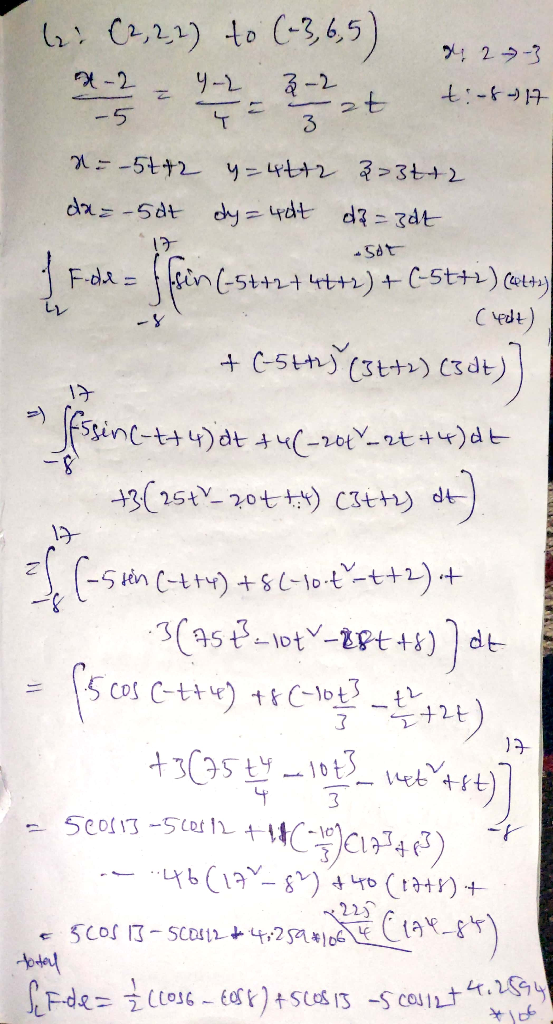Homework Help Question & Answers

# 0/5 points I Previous Answers My Compute the work done by the force F (sin(, y, in moving an obje...0/5 points I Previous Answers My Compute the work done by the force F (sin(, y, in moving an object along the trajectory that is the line segment from (1, 1, ) to (2, 2, 2) followed by the line segment from (2,2, 2) to (-3, 6, 5) when force is measured in Newtons and distance in meters. Submit AnswerIf you have any questions please let me know

Thank you

Please give me thumb up

##### Add Answer of: 0/5 points I Previous Answers My Compute the work done by the force F (sin(, y, in moving an obje...
More Homework Help Questions Additional questions in this topic.

• #### Problem 5 Diagonalize B and compute XA*X-1 to prove this formula for Be, (sections 6.1, 6.2) Bk=15+ 5+-4k has 0 41, Compute also , end sin 0 4 Problem 5 Diagonalize B and compute XA*X-1 to prove...

Need Online Homework Help?

Get FREE EXPERT Answers
WITHIN MINUTES
Related Questions